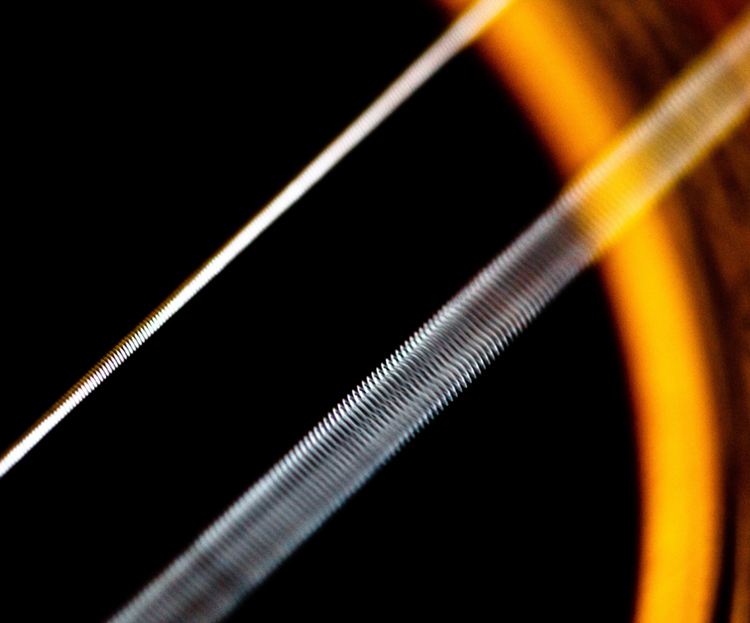Chapter 16 Oscillatory Motion and Waves

# 117 16.2 Period and Frequency in Oscillations

### Summary

• Observe the vibrations of a guitar string.
• Determine the frequency of oscillations.Figure 1. The strings on this guitar vibrate at regular time intervals. (credit: JAR)

When you pluck a guitar string, the resulting sound has a steady tone and lasts a long time. Each successive vibration of the string takes the same time as the previous one. We define periodic motion to be a motion that repeats itself at regular time intervals, such as exhibited by the guitar string or by an object on a spring moving up and down. The time to complete one oscillation remains constant and is called the period$\boldsymbol{T}.$Its units are usually seconds, but may be any convenient unit of time. The word period refers to the time for some event whether repetitive or not; but we shall be primarily interested in periodic motion, which is by definition repetitive. A concept closely related to period is the frequency of an event. For example, if you get a paycheck twice a month, the frequency of payment is two per month and the period between checks is half a month. Frequency$\boldsymbol{f}$is defined to be the number of events per unit time. For periodic motion, frequency is the number of oscillations per unit time. The relationship between frequency and period is

$\boldsymbol{f\:=}$$\boldsymbol{\frac{1}{T}}$

The SI unit for frequency is the cycle per second, which is defined to be a hertz (Hz):

$\boldsymbol{1\textbf{ Hz}=1}$$\boldsymbol{\frac{\textbf{cycle}}{\textbf{sec}}}$$\boldsymbol{\textbf{or }1\textbf{ Hz}=}$$\boldsymbol{\frac{1}{\textbf{s}}}$

A cycle is one complete oscillation. Note that a vibration can be a single or multiple event, whereas oscillations are usually repetitive for a significant number of cycles.

### Example 1: Determine the Frequency of Two Oscillations: Medical Ultrasound and the Period Middle C

We can use the formulas presented in this module to determine both the frequency based on known oscillations and the oscillation based on a known frequency. Let’s try one example of each. (a) A medical imaging device produces ultrasound by oscillating with a period of 0.400 µs. What is the frequency of this oscillation? (b) The frequency of middle C on a typical musical instrument is 264 Hz. What is the time for one complete oscillation?

Strategy

Both questions (a) and (b) can be answered using the relationship between period and frequency. In question (a), the period$\boldsymbol{T}$is given and we are asked to find frequency$\boldsymbol{f}.$In question (b), the frequency$\boldsymbol{f}$is given and we are asked to find the period$\boldsymbol{T}.$

Solution a

1. Substitute$\boldsymbol{0.400\:\mu\textbf{s}}$for$\boldsymbol{T}$in$\boldsymbol{f=\frac{1}{T}}:$
$\boldsymbol{f\:=}$$\boldsymbol{\frac{1}{T}}$$\boldsymbol{=}$$\boldsymbol{\frac{1}{0.400\times10^{-6}\textbf{ s}}}.$

Solve to find

$\boldsymbol{f=2.50\times10^6\textbf{ Hz}}.$

Discussion a

The frequency of sound found in (a) is much higher than the highest frequency that humans can hear and, therefore, is called ultrasound. Appropriate oscillations at this frequency generate ultrasound used for noninvasive medical diagnoses, such as observations of a fetus in the womb.

Solution b

1. Identify the known values:

The time for one complete oscillation is the period$\boldsymbol{T}:$

$\boldsymbol{f=}$$\boldsymbol{\frac{1}{T}}.$
2. Solve for$\boldsymbol{T}:$
$\boldsymbol{T\:=}$$\boldsymbol{\frac{1}{f}}.$
3. Substitute the given value for the frequency into the resulting expression:
$\boldsymbol{T\:=}$$\boldsymbol{\frac{1}{f}}$$\boldsymbol{=}$$\boldsymbol{\frac{1}{264\textbf{ Hz}}}$$\boldsymbol{=}$$\boldsymbol{\frac{1}{264\textbf{ cycles/s}}}$$\boldsymbol{=3.79\times10^{-3}\textbf{ s}=3.79\textbf{ ms}}.$

Discussion

The period found in (b) is the time per cycle, but this value is often quoted as simply the time in convenient units (ms or milliseconds in this case).

### Check your Understanding

1: Identify an event in your life (such as receiving a paycheck) that occurs regularly. Identify both the period and frequency of this event.

# Section Summary

• Periodic motion is a repetitious oscillation.
• The time for one oscillation is the period$\boldsymbol{T}.$
• The number of oscillations per unit time is the frequency$\boldsymbol{f}.$
• These quantities are related by
$\boldsymbol{f\:=}$$\boldsymbol{\frac{1}{T}}.$

### Problems & Exercises

1: What is the period of$\boldsymbol{60.0\textbf{ Hz}}$electrical power?

2: If your heart rate is 150 beats per minute during strenuous exercise, what is the time per beat in units of seconds?

3: Find the frequency of a tuning fork that takes$\boldsymbol{2.50\times10^{-3}\textbf{ s}}$to complete one oscillation.

4: A stroboscope is set to flash every$\boldsymbol{8.00\times10^{-5}\textbf{ s}}.$What is the frequency of the flashes?

5: A tire has a tread pattern with a crevice every 2.00 cm. Each crevice makes a single vibration as the tire moves. What is the frequency of these vibrations if the car moves at 30.0 m/s?

6: Engineering Application

Each piston of an engine makes a sharp sound every other revolution of the engine. (a) How fast is a race car going if its eight-cylinder engine emits a sound of frequency 750 Hz, given that the engine makes 2000 revolutions per kilometer? (b) At how many revolutions per minute is the engine rotating?

## Glossary

period
time it takes to complete one oscillation
periodic motion
motion that repeats itself at regular time intervals
frequency
number of events per unit of time

### Solutions

Check Your Understanding

1: I visit my parents for dinner every other Sunday. The frequency of my visits is 26 per calendar year. The period is two weeks.

Problems & Exercises

1:

16.7 ms

2:

$\boldsymbol{0.400\textbf{ s/beats}}$

3:

400 Hz

4:

12,500 Hz

5:

1.50 kHz

6:

(a) 93.8 m/s

(b)$\boldsymbol{11.3\times10^3\textbf{ rev/min}}$

## LicenseCollege Physics: OpenStax by OpenStax is licensed under a Creative Commons Attribution 4.0 International License, except where otherwise noted.